# ACT Math : How to subtract rational expressions with different denominators

## Example Questions

### Example Question #8 : Expressions

Simplify: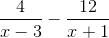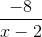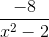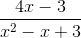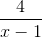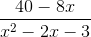Explanation:

The common denominator of these two fractions simply is the product of the two denominators, namely: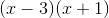Thus, you will need to multiply each fraction's numerator and denominator by the opposite fraction's denominator: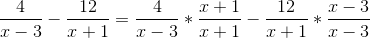Let's first simplify the numerator: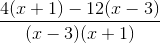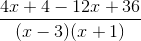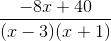, which is the simplest form you will need for this question.

However, the correct answer has the denominator multiplied out. Merely FOIL### Example Question #1 : How To Subtract Rational Expressions With Different Denominators

Simplify: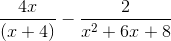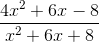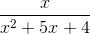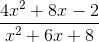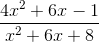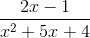Explanation:

Always start the simplification of rational expressions with quadratic denominators by factoring the denominator that has a squared element: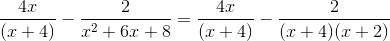Thus, we need to multiply the numerator and denominator of the first term by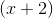: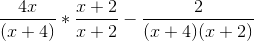Distribute: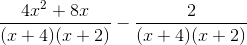This is simply: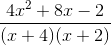You cannot factor the top in any way that would help you to cancel anything. Therefore, the answer stands as it does. You need to FOIL the denominator back out, as your correct answer is in that form:### Example Question #2 : How To Subtract Rational Expressions With Different Denominators

Simplify: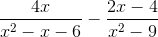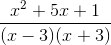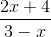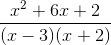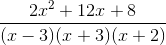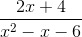Explanation: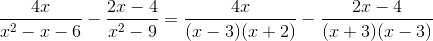Therefore, the common denominator of our fractions is: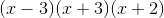This will require you to multiply the first fraction by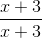and the second by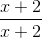. This gives you: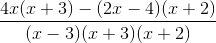Now, FOIL the second member and distribute the first: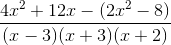Next, distribute the subtraction: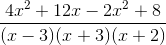Finally, simplify: